Demo problem: Flow of a fluid film down an inclined plane

The two-dimensional flow of a free surface down an inclined plane is a simple exact solution of the Navier–Stokes equations. We describe two different ways of solving the problem using either spines or a pseudo-elastic method to define the bulk mesh motion. Reassuringly, the results are the same irrespective of the method chosen.

# Problem formulation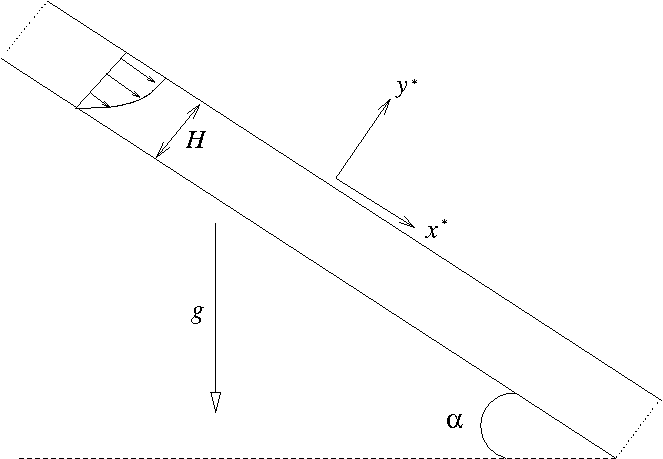A film of incompressible viscous fluid of a given thickness flows down a plane inclined at a prescribed angle to the gravitational field.

Formulating the problem in coordinates tangential (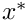) and normal (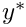) to the plane and assuming that the flow is steady and only in the tangential direction, but independent of the tangential coordinate, reduces the momentum equations to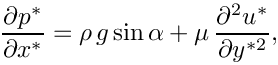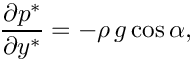where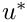is the velocity component tangential to the plane and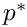is the fluid pressure. Note that the continuity equation is automatically satisfied.

We non-dimensionalise using the only length-scale in the problem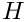, choosing the viscous scale for the pressure and choosing a reference velocity scale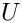: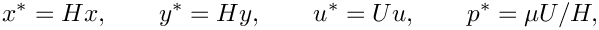and the governing equations become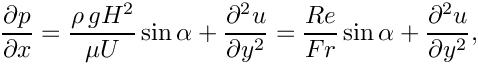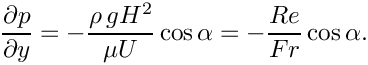The dimensionless grouping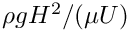represents the ratio of gravitational forces to viscous forces and we choose to identify it as a Reynolds number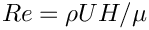divided by a Froude number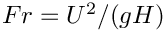.

We proceed by assuming that the flow is driven entirely by the gravitational body force and that there is no additional tangential pressure gradient. Then, integrating the tangential momentum balance twice and using the boundary conditions of no-slip at the plane (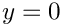)and that the free surface (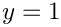) is tangentially stress-free gives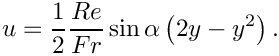Integrating the normal momentum balance and setting the reference external pressure to be zero at the free surface gives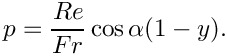Finally, we specify a "natural" velocity scale by setting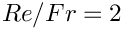, corresponding to the velocity of the free-surface for a vertical film (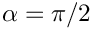).

We shall assess the stability of the flat-film solution by applying a small, short-duration perturbation to the wall velocity and evolving the system in time. If the interface is stable, the perturbation should decay, if not it should grow. A linear stability analysis for this problem was performed by Benjamin (1957) and Yih (1963), who both found that for long waves in the absence of surface tension, the interface was unstable when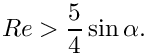(If you read the papers you will see that the Reynolds number was defined such that the average fluid downslope velocity was one; to convert to our Reynolds number, we must multiply by 3/2.)

The figure below shows the time evolution of the interface on a slope of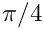for Reynolds numbers of zero (red line) and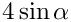(green line). The perturbation wavenumber is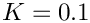and the interface rapidly develops waves that grow as they are convected downslope for the higher Reynolds number, but decay when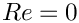.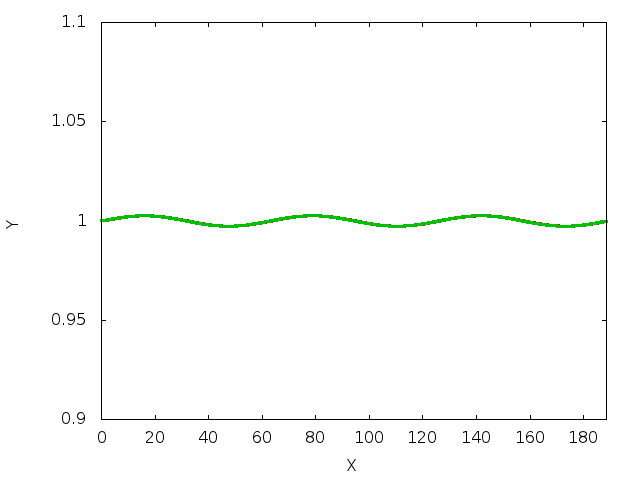Time evolution (or static snapshot at t = 7.5) of the interface shape for Re = 0 (Red) and 4 sin(alpha) (Green).

The decay rate of the interfacial perturbation atis slow, but can be seen in the next figure, which shows the height of the interface at the downstream end of the domain plotted against time. The domain is chosen so that it will contain three waves and the decay or growth of successive crests and troughs can be seen.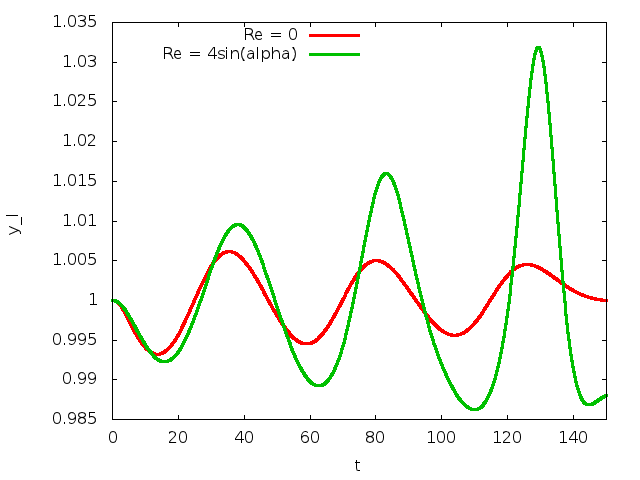Time history of the interface position at the downstream end of the computational domain.

## A note on the boundary conditions

Resolving the above analytic solution in a finite computational domain requires some thought about boundary conditions. We are only ever free to set one pressure value and setting the external pressure to zero fixes the pressure within the fluid. The boundary conditions at the plane are those of no-slip and at the free-surface the usual dynamic and kinematic conditions apply. Nonetheless, we have a number of possibilities for the boundary conditions at the "artificial" upstream and downstream computational boundaries.

• Prescribe periodic boundary conditions.
• Prescribe the velocity profile as a Dirichlet condition at both ends.
• Prescribe the appropriate hydrostatic pressure gradient and zero normal velocity.

We have chosen the last option, in which case the hydrostatic pressure gradient must be consistent with the external pressure. In other words, the pressure must be zero at the free surface (). Changing the external pressure would correspond to changing the film thickness, so the external pressure is directly responsible for enforcing a specific volume constraint, unless. Whenthere is no variation in hydrostatic pressure through the film and its thickness is not specified by the external pressure.

We must also worry about the boundary conditions on the free surface itself and we choose to impose a contact angle condition ofat the upstream end, which ensures that the film remains flat. At the downstream end, we add a line tension term that arises from use of the surface divergence theorem to integrate the contribution of the dynamic boundary condition. This term can be used to enforce contact angle conditions in a weak formulation, but here we simply add the term using the angle calculated from the current position of the free surface.

# Global parameters and functions

The global parameters are the Reynolds number, the dimensionless grouping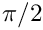, the angle of inclination of the slope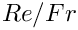, the direction of the gravity vector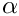and the capillary number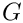, which only influences the dynamics.

{
/// Reynolds number, based on the average velocity within the fluid film
double Re=0.0;
/// The product of Reynolds number and inverse Froude number
/// is set to two in this problem, which gives the free surface velocity
/// to be sin(alpha). [Set to three in order to get the same scale as
/// used by Yih, Benjamin, etc]
double ReInvFr=2.0;
/// Angle of incline of the slope (45 degrees)
double Alpha = 1.0*atan(1.0);
/// The Vector direction of gravity, set in main()
Vector<double> G(2,0.0);
/// The Capillary number
double Ca= 1.0;
double Ca
The Capillary number.
double Alpha
Angle of incline of the slope (45 degrees)
double ReInvFr
The product of Reynolds number and inverse Froude number is set to two in this problem,...
double Re
Reynolds number, based on the average velocity within the fluid film.
Vector< double > G(2, 0.0)
The Vector direction of gravity, set in main()

The hydrostatic pressure field is specified as an applied traction. At the outlet (inlet), the outer unit normal is in the positive (negative)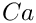direction and so the required traction is given by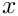(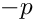),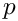These tractions are specified by the two different functions

/// Function that prescribes the hydrostatic pressure field at the outlet
void hydrostatic_pressure_outlet(const double& time, const Vector<double> &x,
const Vector<double> &n,
Vector<double> &traction)
{
traction = ReInvFr*G*(1.0 - x);
traction = 0.0;
}
/// Function that prescribes hydrostatic pressure field at the inlet
void hydrostatic_pressure_inlet(const double& time, const Vector<double> &x,
const Vector<double> &n,
Vector<double> &traction)
{
traction = -ReInvFr*G*(1.0 - x);
traction = 0.0;
}
//end of traction functions
void hydrostatic_pressure_outlet(const double &time, const Vector< double > &x, const Vector< double > &n, Vector< double > &traction)
Function that prescribes the hydrostatic pressure field at the outlet.
void hydrostatic_pressure_inlet(const double &time, const Vector< double > &x, const Vector< double > &n, Vector< double > &traction)
Function that prescribes hydrostatic pressure field at the inlet.

Note that G [ 1 ] is the component of the gravitational body force in the vertical direction, so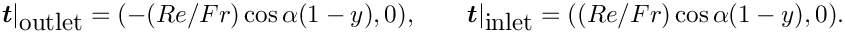.

We must also specify the direction of the normals (directed out of the fluid) to the notional walls that form the inlet and outlet and a contact angle of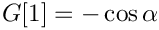that will be used as a boundary condition on the free surface at the upstream end of the domain. In this case the normal to the inlet is in the negative x-direction and the normal to the outlet is in the positive x-direction. The actual value of the Wall_normal vector is set in main()

/// Direction of the wall normal vector (at the inlet)
Vector<double> Wall_normal;
/// Function that specifies the wall unit normal at the inlet
void wall_unit_normal_inlet_fct(const Vector<double> &x,
Vector<double> &normal)
{
normal=Wall_normal;
}
/// Function that specified the wall unit normal at the outlet
void wall_unit_normal_outlet_fct(const Vector<double> &x,
Vector<double> &normal)
{
//Set the normal
normal = Wall_normal;
//and flip the sign
unsigned n_dim = normal.size();
for(unsigned i=0;i<n_dim;++i) {normal[i] *= -1.0;}
}
/// The contact angle that is imposed at the inlet (pi)
double Inlet_Angle = 2.0*atan(1.0);
double Inlet_Angle
The contact angle that is imposed at the inlet (pi)
Vector< double > Wall_normal
Direction of the wall normal vector (at the inlet)
void wall_unit_normal_outlet_fct(const Vector< double > &x, Vector< double > &normal)
Function that specified the wall unit normal at the outlet.
void wall_unit_normal_inlet_fct(const Vector< double > &x, Vector< double > &normal)
Function that specifies the wall unit normal at the inlet.

# The driver code

We start by specifying the constitutive law used to define the mesh motion when pseudo-elastic deformation is used.

//start of main
int main(int argc, char **argv)
{
using namespace Global_Physical_Variables;
//Set the constitutive law for the mesh deformation
int main(int argc, char **argv)
ConstitutiveLaw * Constitutive_law_pt
Constitutive law used to determine the mesh deformation.
double Nu
Pseudo-solid Poisson ratio.

Next, the type of fluid element is chosen according to specified compiler flags

#ifdef CR_ELEMENT
#define FLUID_ELEMENT QCrouzeixRaviartElement<2>
#else
#define FLUID_ELEMENT QTaylorHoodElement<2>
#endif

We then initialise the physical parameters, the Reynolds number and the direction of the gravitational body force, both based on the angle of inclination.

//Initialise physical parameters
//Scale Reynolds number to be independent of alpha.
Re = 4.0/sin(Alpha);
//Set the direction of gravity
G = sin(Alpha);
G = -cos(Alpha);

We also set the direction of the notional wall normal vector.

//The wall normal to the inlet is in the negative x direction
Wall_normal.resize(2);
Wall_normal = -1.0;
Wall_normal = 0.0;

We now create the spine version of the problem, solve the steady problem, assign initial conditions by assuming that the problem has been at the steady state for all previous times, and then evolve the system in time.

//Spine problem
{
//Create the problem
//Prepare the problem for timestepping
//(assume that it's been at the flat-film solution for all previous time)
double dt = 0.1;
problem.assign_initial_values_impulsive(dt);
//Timestep it
problem.timestep(dt,2);
} //End of spine problem
double Length
The length of the domain to fit the desired number of waves.

Finally, exactly the same procedure is performed for the elastic problem

//Elastic problem
{
//Create the problem
PseudoSolidNodeUpdateElement<FLUID_ELEMENT,QPVDElement<2,3> >, BDF<2> >
//Prepare the problem for timestepping
//(assume that it's been at the flat-film solution for all previous time)
double dt = 0.1;
problem.assign_initial_values_impulsive(dt);
//Timestep it
problem.timestep(dt,2);
} //End of elastic problem

# The mesh classes

The base mesh class is the SimpleRectangularQuadMesh: boundary 0 will be the wall; boundary 2 will be the free surface; and the remaining boundaries will be the inlet (3) and outlet (1). Below we shall demonstrate how to convert an existing mesh into a SpineMesh and ElasticMesh suitable for free-surface problems.

## Creating the spine mesh

The SpineInclinedPlaneMesh inherits from the generic SimpleRectangularQuadMesh and adds vertical spines to the Nodes within the mesh in the constructor. Note that the resulting mesh is essentially the same as the SingleLayerSpineMesh, but has a somewhat simpler interface.

/// Create a spine mesh for the problem
//======================================================================
template <class ELEMENT>
public SpineMesh
{
public:
SpineInclinedPlaneMesh(const unsigned &nx, const unsigned &ny,
const double &lx, const double &ly,
TimeStepper* time_stepper_pt) :
(nx,ny,lx,ly,time_stepper_pt), SpineMesh()
{
//Find the number of linear points in the element
unsigned n_p = dynamic_cast<ELEMENT*>(finite_element_pt(0))->nnode_1d();
//Reserve storage for the number of spines
Spine_pt.reserve((n_p-1)*nx + 1);
//Create single pointer to a spine
Spine* new_spine_pt=0;
//Now loop over the elements horizontally
for(unsigned long j=0;j<nx;j++)
{
//In most elements, we don't assign a spine to the last column,
//beacuse that will be done by the next element
unsigned n_pmax = n_p-1;
//In the last element, however, we must assign the final spine
if(j==nx-1) {n_pmax = n_p;}
//Loop over all nodes horizontally
for(unsigned l2=0;l2<n_pmax;l2++)
{
//Create a new spine with unit height and add to the mesh
new_spine_pt=new Spine(1.0);
Spine_pt.push_back(new_spine_pt);
// Get the node
SpineNode* nod_pt=element_node_pt(j,l2);
//Set the pointer to spine
nod_pt->spine_pt() = new_spine_pt;
//Set the fraction
nod_pt->fraction() = 0.0;
// Pointer to the mesh that implements the update fct
nod_pt->spine_mesh_pt() = this;
//Loop vertically along the spine
//Loop over the elements
for(unsigned long i=0;i<ny;i++)
{
//Loop over the vertical nodes, apart from the first
for(unsigned l1=1;l1<n_p;l1++)
{
// Get the node
SpineNode* nod_pt=element_node_pt(i*nx+j,l1*n_p+l2);
//Set the pointer to the spine
nod_pt->spine_pt() = new_spine_pt;
//Set the fraction
nod_pt->fraction()=(double(i)+double(l1)/double(n_p-1))/double(ny);
// Pointer to the mesh that implements the update fct
nod_pt->spine_mesh_pt() = this;
}
}
}
} //End of horizontal loop over elements
} //end of constructor
Create a spine mesh for the problem.
SpineInclinedPlaneMesh(const unsigned &nx, const unsigned &ny, const double &lx, const double &ly, TimeStepper *time_stepper_pt)

In addition, a spine_node_update() function must be provided that determines how the Nodes move as functions of the Spines.

/// General node update function implements pure virtual function
/// defined in SpineMesh base class and performs specific node update
/// actions: along vertical spines
virtual void spine_node_update(SpineNode* spine_node_pt)
{
//Get fraction along the spine
double W = spine_node_pt->fraction();
//Get spine height
double H = spine_node_pt->h();
//Set the value of y
spine_node_pt->x(1) = W*H;
}

## Creating the ElasticMesh

The ElasticInclinedPlaneMesh inherits from the SimpleRectangularQuadMesh and the undeformed (reference) configuration is set to be the current position of the Nodes.

/// Create an Elastic mesh for the problem
//======================================================================
template <class ELEMENT>
public SolidMesh
{
//Public functions
public:
ElasticInclinedPlaneMesh(const unsigned &nx, const unsigned &ny,
const double &lx, const double &ly,
TimeStepper* time_stepper_pt) :
{
//Make the current configuration the undeformed one
set_lagrangian_nodal_coordinates();
}
};
Create an Elastic mesh for the problem.
ElasticInclinedPlaneMesh(const unsigned &nx, const unsigned &ny, const double &lx, const double &ly, TimeStepper *time_stepper_pt)

Note that the specification of the ElasticMesh is much simpler than that of a SpineMesh because no decision needs to be taken about how to describe the motion using Spines.

# The problem classes

## The generic problem

For ease of exposition, all generic functionality is included in the InclinedPlaneProblem class, which is templated by the bulk ELEMENT and the INTERFACE_ELEMENT. The class includes storage for the different sub-meshes: Bulk, the Traction elements associated with the inlet and outlet, the (free) Surface elements and the point elements associated with the ends of the interface. In addition, a string Output_prefix is used to distinguish between the output files from different formulations.

/// Generic problem class that will form the base class for both
/// spine and elastic mesh-updates of the problem.
/// Templated by the bulk element and interface element types
//====================================================================
template<class ELEMENT, class INTERFACE_ELEMENT>
class InclinedPlaneProblem : public Problem
{
protected:
/// Bulk fluid mesh
Mesh* Bulk_mesh_pt;
/// Mesh for the traction elements that are added at inlet and outlet
/// Mesh for the free surface elements
/// Mesh for the point elements at each end of the free surface
/// Prefix for output files
std::string Output_prefix;
Generic problem class that will form the base class for both spine and elastic mesh-updates of the pr...
Mesh * Bulk_mesh_pt
Bulk fluid mesh.
std::string Output_prefix
Prefix for output files.
Mesh * Traction_mesh_pt
Mesh for the traction elements that are added at inlet and outlet.
Mesh * Surface_mesh_pt
Mesh for the free surface elements.
Mesh * Point_mesh_pt
Mesh for the point elements at each end of the free surface.

The time-dependent perturbation is introduced in the function actions_before_implicit_timestep(), which sets the vertical velocity on the wall (boundary 0)void actions_before_implicit_timestep()
{
double time = this->time_pt()->time();
//Now add a temporary sinusoidal suction and blowing to the base
//Amplitude of the perturbation
double epsilon = 0.01;
//Loop over the nodes on the base
unsigned n_node = this->Bulk_mesh_pt->nboundary_node(0);
for(unsigned n=0;n<n_node;n++)
{
Node* nod_pt = this->Bulk_mesh_pt->boundary_node_pt(0,n);
double arg = Global_Physical_Variables::K*nod_pt->x(0);
double value = sin(arg)*epsilon*time*exp(-time);
nod_pt->set_value(1,value);
}
} //end_of_actions_before_implicit_timestep
double K
Set the wavenumber.

The function make_traction_elements() creates NavierStokesTractionElement s adjacent to the mesh boundaries 3 (the inlet) and 1 (the inlet). These elements are added to the Mesh Traction_mesh_pt, which is itself constructed in the function and pointers to the appropriate traction functions are assigned.

/// Function to add the traction boundary elements to boundaries
/// 3(inlet) and 1(outlet) of the mesh
void make_traction_elements()
{
//Create a new (empty mesh)
Traction_mesh_pt = new Mesh;
//Inlet boundary conditions (boundary 3)
{
unsigned b = 3;
//Find the number of elements adjacent to mesh boundary
unsigned n_boundary_element = Bulk_mesh_pt->nboundary_element(b);
//Loop over these elements and create the traction elements
for(unsigned e=0;e<n_boundary_element;e++)
{
NavierStokesTractionElement<ELEMENT> *surface_element_pt =
new NavierStokesTractionElement<ELEMENT>
(Bulk_mesh_pt->boundary_element_pt(b,e),
Bulk_mesh_pt->face_index_at_boundary(b,e));
//Add the elements to the mesh
//Set the traction function
surface_element_pt->traction_fct_pt() =
}
}
//Outlet boundary conditions (boundary 1)
{
unsigned b=1;
//Find the number of elements adjacent to mesh boundary
unsigned n_boundary_element = Bulk_mesh_pt->nboundary_element(b);
//Loop over these elements and create the traction elements
for(unsigned e=0;e<n_boundary_element;e++)
{
NavierStokesTractionElement<ELEMENT> *surface_element_pt =
new NavierStokesTractionElement<ELEMENT>
(Bulk_mesh_pt->boundary_element_pt(b,e),
Bulk_mesh_pt->face_index_at_boundary(b,e));
//Add the elements to the mesh
//Set the traction function
surface_element_pt->traction_fct_pt() =
}
}
} //end of make_traction_elements

The function make_free_surface_elements() creates the appropriate INTERFACE_ELEMENTs adjacent to the free surface (boundary 2), sets the capillary number and also creates free-surface boundary elements at the left- and right-hand ends of the interface. If these "point" elements are not included then the surface tension is not applied correctly at the edges of the domain. The contact angle is set to be the value Inlet_Angle at the left-hand edge of the domain.

//Make the free surface elements on the top surface
void make_free_surface_elements()
{
//Create the (empty) meshes
Surface_mesh_pt = new Mesh;
Point_mesh_pt = new Mesh;
//The free surface is on the boundary 2
unsigned b = 2;
unsigned n_boundary_element = Bulk_mesh_pt->nboundary_element(b);
//Loop over the elements and create the appropriate interface elements
for(unsigned e=0;e<n_boundary_element;e++)
{
INTERFACE_ELEMENT *surface_element_pt =
new INTERFACE_ELEMENT
(Bulk_mesh_pt->boundary_element_pt(b,e),
Bulk_mesh_pt->face_index_at_boundary(b,e));
//Assign the capillary number to the free surface
surface_element_pt->ca_pt() =
//Make a point element from left-hand side of the
//first surface element (note that this relies on knowledge of
//the element order within the mesh)
if(e==0)
{
FluidInterfaceBoundingElement* point_element_pt =
surface_element_pt->make_bounding_element(-1);
//Add element to the point mesh
//Set the capillary number
point_element_pt->ca_pt() = &Global_Physical_Variables::Ca;
//Set the wall normal
point_element_pt->wall_unit_normal_fct_pt() =
//Set the contact angle (using the strong version of the constraint)
point_element_pt->set_contact_angle(
}
//Make another point element from the right-hand side of the
//last surface element (note that this relies on knowledge of
//the element order within the mesh)
if(e==n_boundary_element-1)
{
FluidInterfaceBoundingElement* point_element_pt =
surface_element_pt->make_bounding_element(1);
//Set the capillary number
point_element_pt->ca_pt() = &Global_Physical_Variables::Ca;
// Set the function that specifies the wall normal
point_element_pt->wall_unit_normal_fct_pt() =
}
}
} //end of make_free_surface_elements

The function complete_build() assigns physical parameters to the fluid elements, sets the boundary conditions and assigns equation numbers.

void complete_build()
{
using namespace Global_Physical_Variables;
//Complete the build of the fluid elements by passing physical parameters
//Find the number of bulk elements
unsigned n_element = Bulk_mesh_pt->nelement();
//Loop over all the fluid elements
for(unsigned e=0;e<n_element;e++)
{
//Cast to a fluid element
ELEMENT *temp_pt = dynamic_cast<ELEMENT*>(Bulk_mesh_pt->element_pt(e));
//Set the Reynolds number
temp_pt->re_pt() = &Re;
//The Strouhal number is 1, so ReSt = Re
temp_pt->re_st_pt() = &Re;
//Set the Reynolds number / Froude number
temp_pt->re_invfr_pt() = &ReInvFr;
//Set the direction of gravity
temp_pt->g_pt() = &G;
}
//------------Set the boundary conditions for this problem----------
{
//Determine whether we are solving an elastic problem or not
bool elastic = false;
if(dynamic_cast<SolidNode*>(Bulk_mesh_pt->node_pt(0))) {elastic=true;}
//Loop over the bottom of the mesh (the wall of the channel)
unsigned n_node = Bulk_mesh_pt->nboundary_node(0);
for(unsigned j=0;j<n_node;j++)
{
//Pin the u- and v- velocities
Bulk_mesh_pt->boundary_node_pt(0,j)->pin(0);
Bulk_mesh_pt->boundary_node_pt(0,j)->pin(1);
//If we are formulating the elastic problem pin both positions
//of nodes
if(elastic)
{
static_cast<SolidNode*>(Bulk_mesh_pt->boundary_node_pt(0,j))
->pin_position(0);
static_cast<SolidNode*>(Bulk_mesh_pt->boundary_node_pt(0,j))
->pin_position(1);
}
}
//Loop over the inlet and set the Dirichlet condition
//of no vertical velocity
n_node = Bulk_mesh_pt->nboundary_node(3);
for(unsigned j=0;j<n_node;j++)
{
Bulk_mesh_pt->boundary_node_pt(3,j)->pin(1);
//If elastic pin horizontal position of nodes
if(elastic)
{
static_cast<SolidNode*>(Bulk_mesh_pt->boundary_node_pt(3,j))
->pin_position(0);
}
}
//Loop over the outlet and set the Dirichlet condition
//of no vertical velocity
n_node = Bulk_mesh_pt->nboundary_node(1);
for(unsigned j=0;j<n_node;j++)
{
Bulk_mesh_pt->boundary_node_pt(1,j)->pin(1);
//If elastic pin horizontal position
if(elastic)
{
static_cast<SolidNode*>(Bulk_mesh_pt->boundary_node_pt(1,j))
->pin_position(0);
}
}
}
//Attach the boundary conditions to the mesh
std::cout << assign_eqn_numbers() << " in the main problem" << std::endl;
} //end of complete_build

Note that boundary conditions for the nodal positions in the pseudo-elastic formulation are specified by testing whether the Nodes are SolidNodes. In this case, the Nodes on the inlet and outlet boundaries are constrained to remain at the same horizontal position and the Nodes on the plane wall are fixed.

The function solve_steady() initialises the velocity of at all Nodes to the flat-film solution, solves the steady equations and writes the solution to a file.

{
using namespace Global_Physical_Variables;
//Initially set all nodes to the Nusselt flat-film solution
{
unsigned n_node = Bulk_mesh_pt->nnode();
for(unsigned n=0;n<n_node;n++)
{
double y = Bulk_mesh_pt->node_pt(n)->x(1);
//Top row
Bulk_mesh_pt->node_pt(n)->set_value(0,0.5*ReInvFr*sin(Alpha)*(2.0*y - y*y));
}
}
//Output the full flow field
std::string filename = Output_prefix;;
filename.append("_output.dat");
ofstream file(filename.c_str());
Bulk_mesh_pt->output(file,5);
file.close();

Finally, the function timestep() takes a number of fixed timesteps writing vertical positions and the time to a trace file and writing the complete flow field to disk after a given number of timesteps.

timestep(const double &dt, const unsigned &n_tsteps)
{
//Need to use the Global variables here
using namespace Global_Physical_Variables;
//Open an output file
std::string filename = Output_prefix;
filename.append("_time_trace.dat");
ofstream trace(filename.c_str());
//Counter that will be used to output the full flowfield
//at certain timesteps
int counter=0;
//Initial output of the time and the value of the vertical position at the
//left and right-hand end of the free surface
trace << time_pt()->time() << " "
<< Bulk_mesh_pt->boundary_node_pt(2,0)->value(1)
<< " "
<< Bulk_mesh_pt->
boundary_node_pt(2, Bulk_mesh_pt->nboundary_node(2)-1)->x(1)
<< " "
<< std::endl;
//Loop over the desired number of timesteps
for(unsigned t=1;t<=n_tsteps;t++)
{
//Increase the counter
counter++;
cout << std::endl;
cout << "--------------TIMESTEP " << t<< " ------------------" << std::endl;
//Take a timestep of size dt
//Uncomment to get full solution output
if(counter==2) //Change this number to get output every n steps
{
std::ofstream file;
std::ostringstream filename;
filename << Output_prefix << "_step" << Re << "_" << t << ".dat";
file.open(filename.str().c_str());
Bulk_mesh_pt->output(file,5);
file.close();
counter=0;
}
//Always output the interface
{
std::ofstream file;
std::ostringstream filename;
filename << Output_prefix << "_interface_" << Re << "_" << t << ".dat";
file.open(filename.str().c_str());
Surface_mesh_pt->output(file,5);
file.close();
}
//Output the time and value of the vertical position of the free surface
//at the left- and right-hand ends
trace << time_pt()->time() << " "
<< Bulk_mesh_pt->boundary_node_pt(2,0)->x(1) << " "
<<
Bulk_mesh_pt->
boundary_node_pt(2,Bulk_mesh_pt->nboundary_node(2)-1)->x(1) << " "
<< std::endl;
}
} //end of timestep

## The spine-based formulation

The class SpineInclinedPlaneProblem inherits from the generic InclinedPlaneProblem class and requires only minor modification. The constructor sets the string Output_prefix, builds a timestepper, builds the specific SpineMesh, creates the appropriate FaceElements, adds all sub-meshes to the Problem, builds the global mesh and then calls InclinedPlaneProblem::complete_build().

SpineInclinedPlaneProblem(const unsigned &nx, const unsigned &ny,
const double &length):
InclinedPlaneProblem<ELEMENT,SpineLineFluidInterfaceElement<ELEMENT> >
(nx,ny,length)
{
//Set the name
this->Output_prefix = "spine";
//Create our one and only timestepper, with adaptive timestepping
//Create the bulk mesh
this->Bulk_mesh_pt = new SpineInclinedPlaneMesh<ELEMENT>(
nx,ny,length,1.0,this->time_stepper_pt());
//Create the traction elements
this->make_traction_elements();
//Create the free surface elements
this->make_free_surface_elements();
//Add all sub meshes to the problem
//Create the global mesh
this->build_global_mesh();
//Complete the build of the problem
this->complete_build();
}

In a spine-based formulation, the nodal positions must be updated after every Newton step, which is achieved by overloading the function Problem::actions_before_newton_convergence_check()

/// Spine heights/lengths are unknowns in the problem so their
/// values get corrected during each Newton step. However,
/// changing their value does not automatically change the
/// nodal positions, so we need to update all of them
void actions_before_newton_convergence_check()
{this->Bulk_mesh_pt->node_update();}

We also specify a destructor to clean up memory allocated by the class.

## The pseudo-solid-based formulation

The class ElasticInclinedPlaneProblem inherits from the generic InclinedPlaneProblem class and also requires only minor modification. The constructor sets the string Output_prefix, builds a timestepper, builds the specific SolidMesh, sets the constitutive law for the bulk elements, creates the appropriate FaceElements, adds all sub-meshes to the Problem, builds the global mesh and then calls InclinedPlaneProblem::complete_build()

ElasticInclinedPlaneProblem(const unsigned &nx, const unsigned &ny,
const double &length) :
InclinedPlaneProblem<ELEMENT,ElasticLineFluidInterfaceElement<ELEMENT> >
(nx,ny,length)
{
//Set the name
this->Output_prefix = "elastic";
//Create our one and only timestepper, with adaptive timestepping
//Create the bulk mesh
this->Bulk_mesh_pt = new ElasticInclinedPlaneMesh<ELEMENT>(
nx,ny,length,1.0,this->time_stepper_pt());
//Set the consititutive law for the elements
unsigned n_element = this->Bulk_mesh_pt->nelement();
//Loop over all the fluid elements
for(unsigned e=0;e<n_element;e++)
{
//Cast to a fluid element
ELEMENT *temp_pt = dynamic_cast<ELEMENT*>(
this->Bulk_mesh_pt->element_pt(e));
//Set the constitutive law
temp_pt->constitutive_law_pt() =
}
//Create the traction elements
this->make_traction_elements();
//Create the free surface element
this->make_free_surface_elements();
//Add all sub meshes to the problem
//Create the global mesh
this->build_global_mesh();
//Complete the rest of the build
this->complete_build();
} //end of constructor

In a pseudo-solid formulation, it is advantageous to reset the undeformed configuration after every timestep (an updated Lagrangian formulation). Hence, the Problem::actions_after_implicit_timestep() function is overloaded

void actions_after_implicit_timestep()
{
//Now loop over all the nodes and reset their Lagrangian coordinates
unsigned n_node = this->Bulk_mesh_pt->nnode();
for(unsigned n=0;n<n_node;n++)
{
//Cast node to an elastic node
SolidNode* temp_pt =
static_cast<SolidNode*>(this->Bulk_mesh_pt->node_pt(n));
for(unsigned j=0;j<2;j++) {temp_pt->xi(j) = temp_pt->x(j);}
}
} //end of actions_after_implicit_timestep

We also specify a destructor to clean up memory allocated by the class.

# Exercises

1. Confirm that the steady solution agrees with the exact solution.
2. Investigate what happens when the angle is varied. What happens when the angle is set to zero? What happens when the angle is set to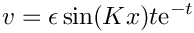?
3. What happens if the hydrostatic pressure boundary conditions are not applied?
4. How does the stability of the system to the perturbation change with angle,and? Are the results in agreement with the theoretical predictions?
5. Are the results independent of the length of the domain?
6. Compare the spine-based and pseudo-elastic-based formulations? What is the same and what is different? Which method do you prefer?